# Copy the UsedRange of each sheet into one sheet using VBA in Microsoft Excel

In case you want to copy the used range of each worksheet into Master sheet then you should read this article. We will use VBA code to copy the data from each worksheet & then paste in another sheet without overwriting.

The macro will add a sheet with the name Master to your workbook and will copy the cells from every sheet in your workbook in this worksheet.

The first macro does a normal copy and the second macro copies the Values. The macro’s subs use the functions below; the macro’s won’t work without the functions.

Following are the snapshot of data from Sheet1 & Sheet2: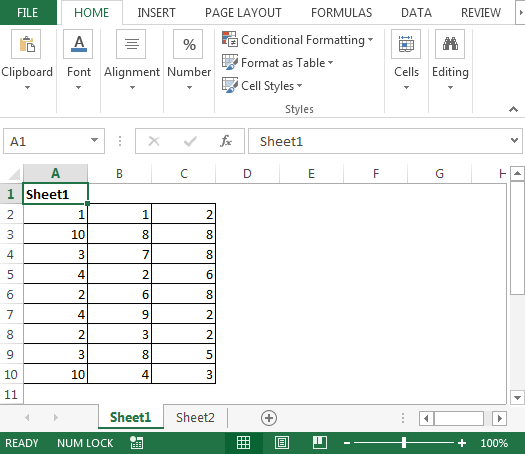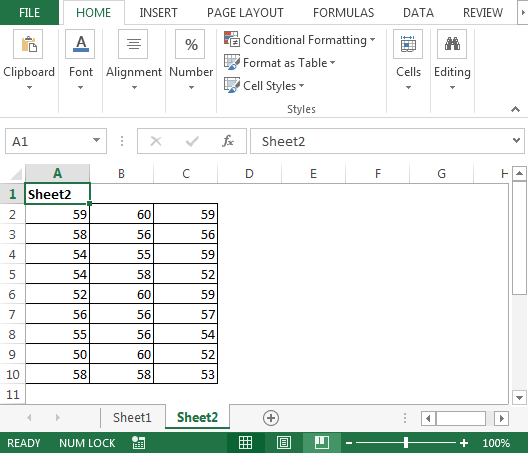We need to follow the below steps to launch VB editor:

• Click on Developer tab
• From Code group, select Visual Basic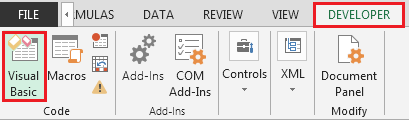• Copy the below code in the standard module

```Sub CopyUsedRange()
Dim sh As Worksheet
Dim DestSh As Worksheet
Dim Last As Long
If SheetExists("Master") = True Then
MsgBox "The sheet Master already exist"
Exit Sub
End If
Application.ScreenUpdating = False
DestSh.Name = "Master"
For Each sh In ThisWorkbook.Worksheets
If sh.Name <> DestSh.Name Then
If sh.UsedRange.Count > 1 Then
Last = LastRow(DestSh)
sh.UsedRange.Copy DestSh.Cells(Last + 1, 1)
End If
End If
Next
Application.ScreenUpdating = True
End Sub

Sub CopyUsedRangeValues()
Dim sh As Worksheet
Dim DestSh As Worksheet
Dim Last As Long
If SheetExists("Master") = True Then
MsgBox "The sheet Master already exist"
Exit Sub
End If
Application.ScreenUpdating = False
DestSh.Name = "Master"
For Each sh In ThisWorkbook.Worksheets
If sh.Name <> DestSh.Name Then
If sh.UsedRange.Count > 1 Then
Last = LastRow(DestSh)
With sh.UsedRange
DestSh.Cells(Last + 1, 1).Resize(.Rows.Count, _
.Columns.Count).Value = .Value
End With
End If
End If
Next
Application.ScreenUpdating = True
End Sub

Function LastRow(sh As Worksheet)
On Error Resume Next
LastRow = sh.Cells.Find(What:="*", _
After:=sh.Range("A1"), _
Lookat:=xlPart, _
LookIn:=xlFormulas, _
SearchOrder:=xlByRows, _
SearchDirection:=xlPrevious, _
MatchCase:=False).Row
On Error GoTo 0
End Function

Function Lastcol(sh As Worksheet)
On Error Resume Next
Lastcol = sh.Cells.Find(What:="*", _
After:=sh.Range("A1"), _
Lookat:=xlPart, _
LookIn:=xlFormulas, _
SearchOrder:=xlByColumns, _
SearchDirection:=xlPrevious, _
MatchCase:=False).Column
On Error GoTo 0
End Function

Function SheetExists(SName As String, _
Optional ByVal WB As Workbook) As Boolean
On Error Resume Next
If WB Is Nothing Then Set WB = ThisWorkbook
SheetExists = CBool(Len(Sheets(SName).Name))
End Function

```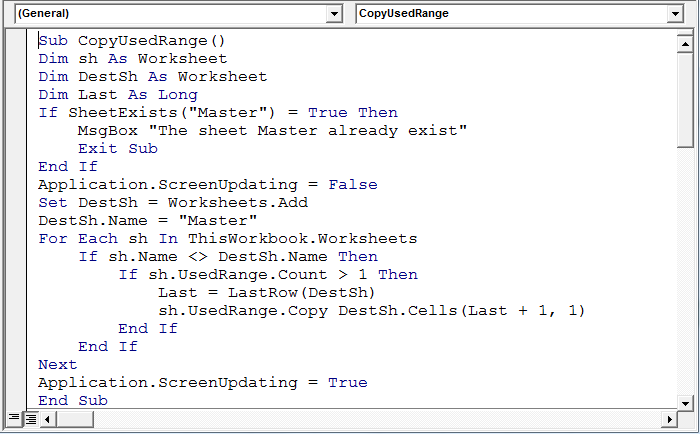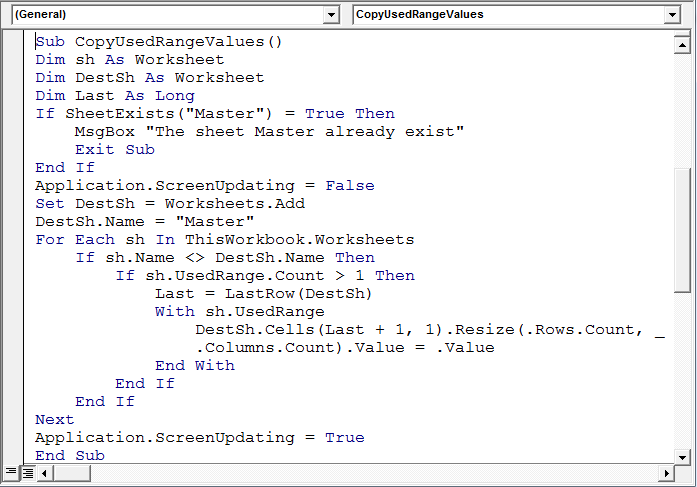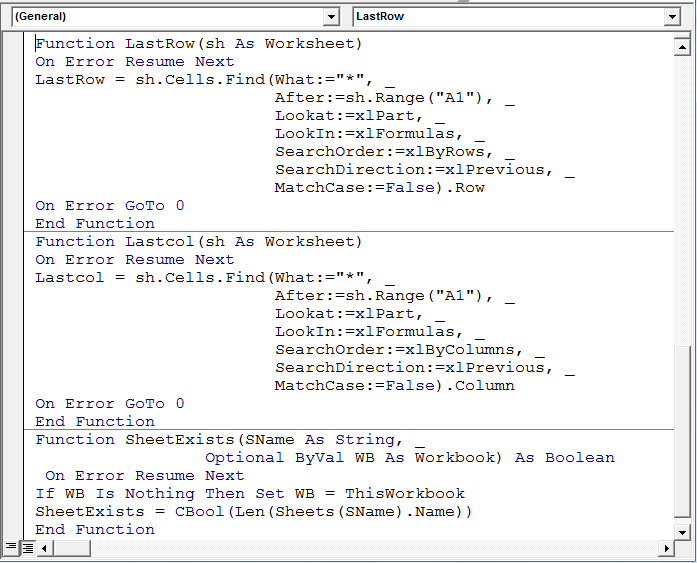Now, the macro code is set; we will run the macro “CopyUsedRange” & it will insert a new sheet “Master” & copy the data from each sheet.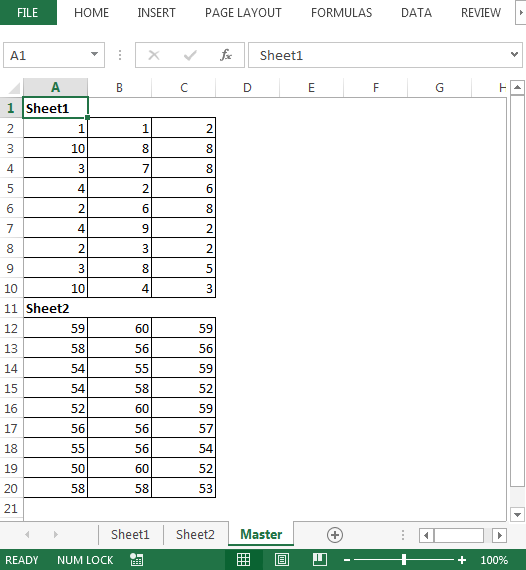Conclusion: Copying data from multiple sheets is a manual task; however; with the above code, we can consolidate data with a single click on a macro.